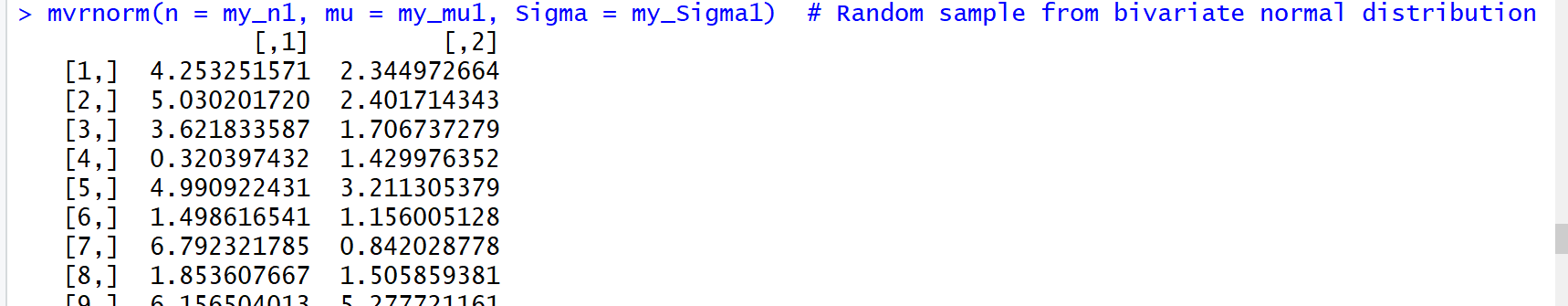# Simulate Bivariate & Multivariate Normal Distribution in R (2 Examples)

This post explains how to draw a random bivariate and multivariate normal distribution in the R programming language.

Let’s do this.

## Example 1: Bivariate Normal Distribution in R

Example 1 explains how to generate a random bivariate normal distribution in R.

First, we have to install and load the MASS package to R:

```install.packages("MASS")                            # Install MASS package

In case we want to create a reproducible set of random numbers, we also have to set a seed:

`set.seed(98989)                                     # Set seed for reproducibility`

Then, we have to specify the data setting that we want to create. The following R code specifies the sample size of random numbers that we want to draw (i.e. 1000), the means of our two normal distributions (i.e. 5 and 2), and the variance-covariance matrix of our two variables:

```my_n1 <- 1000                                       # Specify sample size
my_mu1 <- c(5, 2)                                   # Specify the means of the variables
my_Sigma1 <- matrix(c(10, 5, 3, 7),                 # Specify the covariance matrix of the variables
ncol = 2)```

After specifying all our input arguments, we can apply the mvrnorm function of the MASS package as follows:

`mvrnorm(n = my_n1, mu = my_mu1, Sigma = my_Sigma1)  # Random sample from bivariate normal distribution`Figure 1: Bivariate Random Numbers with Normal Distribution.

Figure 1 illustrates the RStudio output of our previous R syntax. The R code returned a matrix with two columns, whereby each of these columns represents one of the normal distributions.

## Example 2: Multivariate Normal Distribution in R

In Example 2, we will extend the R code of Example 1 in order to create a multivariate normal distribution with three variables. As in Example 1, we need to specify the input arguments for the mvrnorm function. However, this time we are specifying three means and a variance-covariance matrix with three columns:

```my_n2 <- 1000                                       # Specify sample size
my_mu2 <- c(5, 2, 8)                                # Specify the means of the variables
my_Sigma2 <- matrix(c(10, 5, 2, 3, 7, 1, 1, 8, 3),  # Specify the covariance matrix of the variables
ncol = 3)```

We can now apply the mvrnorm as we already did in Example 1:

`mvrnorm(n = my_n2, mu = my_mu2, Sigma = my_Sigma2)  # Random sample from bivariate normal distribution`Figure 2: Multivariate Random Numbers with Normal Distribution.

Figure 2 illustrates the output of the R code of Example 2. This time, R returned a matrix consisting of three columns, whereby each of the three columns represents one normally distributed variable.

## Video, Further Resources & Summary

Do you need further information on the contents of this article? Then you could have a look at the following video that I have published on my YouTube channel. In the video, I explain the topics of this tutorial:

You could also have a look at the other tutorials on probability distributions and the simulation of random numbers in R:

Besides that, you may read some of the other tutorials that I have published on my website:

Summary: In this R programming tutorial you learned how to simulate bivariate and multivariate normally distributed probability distributions. In case you have any additional questions, please tell me about it in the comments section below.

Subscribe to the Statistics Globe Newsletter